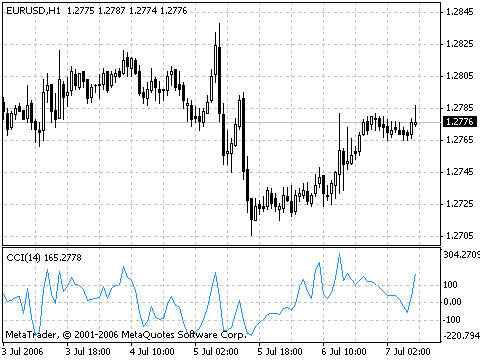Commodity Channel Index, CCI

Commodity Channel Index, CCI

23003
0
SHAREA useful indicator that can make an interesting trade trigger by itself or part of a broader technical trading approach.

Commodity Channel Index (CCI) indicator measures the deviation of the commodity price from its average statistical price.

High values of the index point out that the price is unusually high being compared with the average one, and low values show that the price is too low.

In spite of its name, the Commodity Channel Index can be applied for any financial instrument, and not only for the commodities.There are two basic techniques of using Commodity Channel Index:

1. Finding the divergences
The divergence appears when the price reaches a new maximum, and Commodity Channel Index can not grow above the previous maximums. This classical divergence is normally followed by the price correction.
2. As an indicator of overbuying/overselling
Commodity Channel Index usually varies in the range of ±100. Values above +100 inform about overbuying state (and about a probability of correcting decay), and the values below 100 inform about the overselling state (and about a probability of correcting increase).

Calculation:

1. To find a Typical Price. You need to add the HIGH, the LOW, and the CLOSE prices of each bar and then divide the result by 3.

TP = (HIGH + LOW +CLOSE)/3

2. To calculate the n-period Simple Moving Average of typical prices.

SMA(TP, N) = SUM[TP, N]/N

3. To subtract the received SMA(TP, N) from Typical Prices.

D = TP — SMA(TP, N)

4. To calculate the n-period Simple Moving Average of absolute D values.

SMA(D, N) = SUM[D, N]/N

5. To multiply the received SMA(D, N) by 0,015.

M = SMA(D, N) * 0,015

6. To divide M by D

CCI = M/D

where:

• SMA — Simple Moving Average;
• N — number of periods, used for calculation.

Commodity Channel Index, CCI is a Metatrader 4 (MT4) indicator and the essence of the forex indicator is to transform the accumulated history data.

Commodity Channel Index, CCI provides for an opportunity to detect various peculiarities and patterns in price dynamics which are invisible to the naked eye.

Based on this information, traders can assume further price movement and adjust their strategy accordingly.

How to install Commodity Channel Index, CCI.mq4?

• Copy Commodity Channel Index, CCI.mq4 to your Metatrader Directory / experts / indicators /
• Select Chart and Timeframe where you want to test your indicator
• Right click on Commodity Channel Index, CCI.mq4
• Attach to a chart
• Modify settings or press ok
• Indicator Commodity Channel Index, CCI.mq4 is available on your Chart

How to remove Commodity Channel Index, CCI.mq4 from your Metatrader 4 Chart?

• Select the Chart where is the Indicator running in your Metatrader Client
• Right click into the Chart
• “Indicators list”
• Select the Indicator and delete